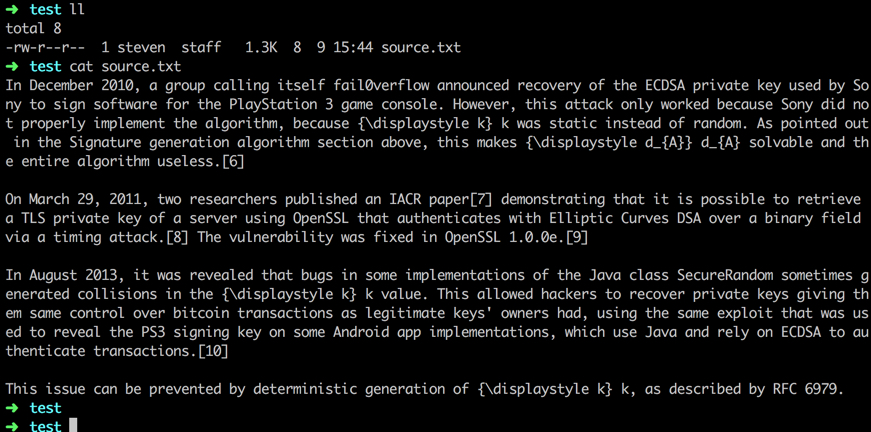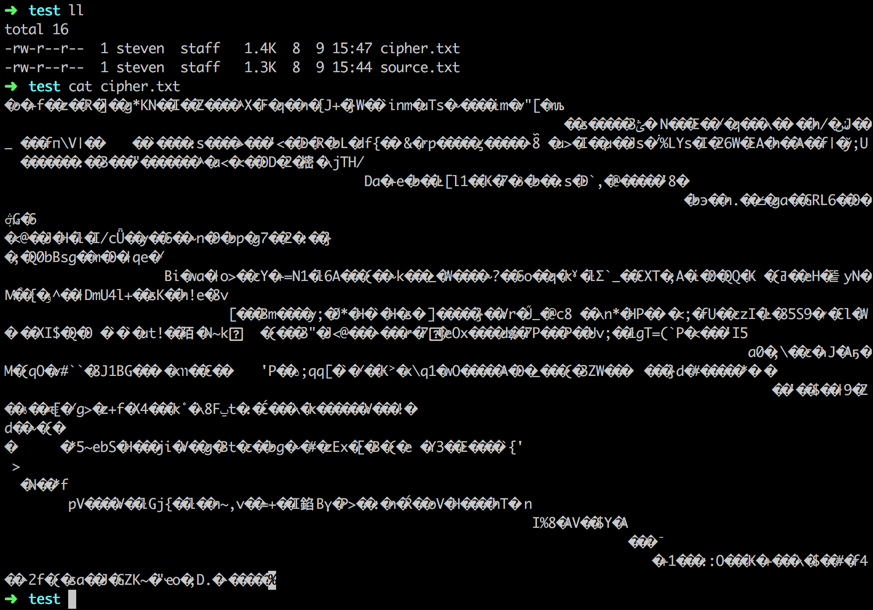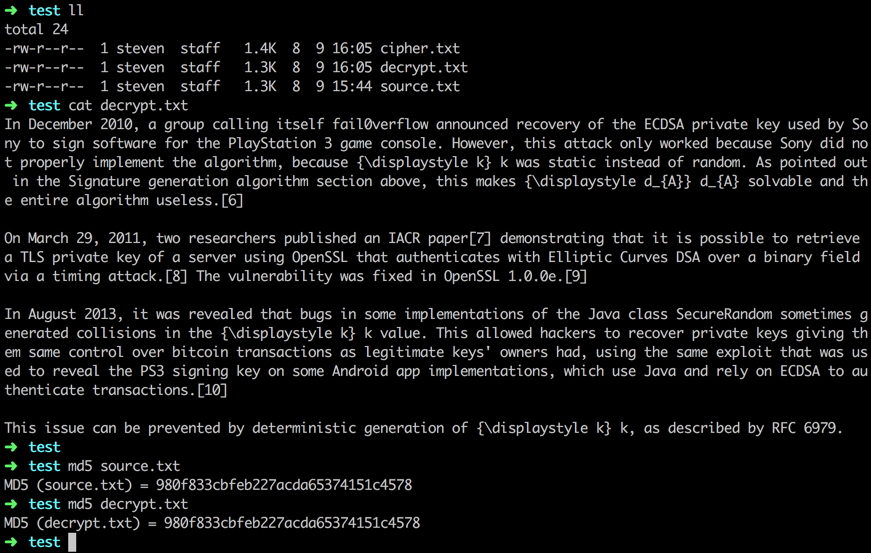# 密钥对的生成

ECDSA(Elliptic Curve Digital Signature Algorithm) 是使用 ECC(Elliptic Curve Cryptography) 椭圆曲线加密算法对 DSA 数字签名算法的模拟，ECDSA 是 1999 年成为 ANSI 标准，并于 2000 年成为 IEEE 和 NIST 标准。与普通的离散对数问题（Discrete Logarithm Problem DLP）和大数分解问题（Integer Factorization Problem IFP）不同，椭圆曲线离散对数问题（Elliptic Curve Discrete Logarithm Problem ECDLP）没有亚指数时间的解决方法。因此椭圆曲线密码的单位比特强度要高于其他公钥体制。

secp256k1 标准也是由美国国家标准和技术研究院 NIST 设立，secp256k1 的一些细节参考

# 示例

IESParameterSpec 为 IES 的算法引擎参数设置，这里的三个参数分别为：

• derivation: 可选，用于 KDF 的推导向量；
• encoding: 可选，用于 KDF 的编码向量；
• macKeySize: 设置 MAC 的 key size，这里设置为 64 个 bits；

KDF(Key Derivation Function) derives one or more secret keys from a secret value such as a master key, a password, or a passphrase using a pseudorandom function.# 常见术语

• EC, Elliptic Curve, 椭圆曲线
• ECC, Elliptic Curve Cryptography, 椭圆曲线密码学
• ECDSA, Elliptic Curve Digital Signature Algorithm, 椭圆曲线数字签名算法
• DH, Diffie-Hellman Key Exchange, Diffie-Hellman 密钥交换
• ECDH, Elliptic Curve Diffie-Hellman Key Exchange, 椭圆曲线 Diffie-Hellman 密钥交换
• IES, Integrated Encryption Schema, 集成加密框架
• ECIES, Elliptic Curve Integrated Encryption Schema, 椭圆曲线集成加密框架
• KDF, Key Derivation Function, 密钥(私钥)生成函数